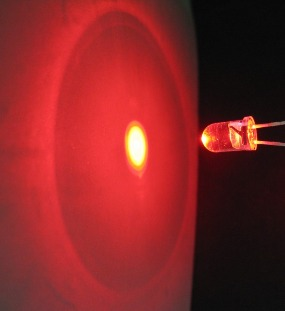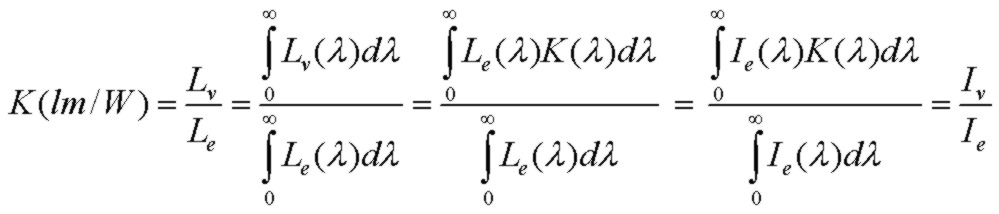# LED: Radiant and Luminous Intensity

July 20, 2012Light emitting diodes (LED) have output intensities that are specified in two types of units: radiant intensity (mW/sr = milliWatts per steradian) and luminous intensity (photometric units) (mcd = millicandelas = millilumens per steradian = mlm/sr) at a typical forward current (e.g. 20 mA) and at the peak of the forward emission radiation pattern. The steradian (sr) is the unit of solid-angle measurement. Some LED specification sheets provide a plot showing how the radiation intensity pattern depends on direction angle θ. A typical radiation field from a high-radiance red LED is shown to the right. The bright center spot is the main radiation field with an intensity half-width of about 15 degrees. Note that if the LED emitting source was a planar Lambertian emitter (perfectly diffuse source), the half-intensity angle would be 60 degrees (Lambert's cosine law). The design of real LEDs (chip design and packaging reflectors etc.) however usually places more radiant intensity into a narrower radiation pattern.

Usually LEDs emitting in the visible (e.g. red, green, white) use the luminous (photometric) units while infrared LEDs use radiant units. However, photodiode sensitivity is often specified in terms of radiant units (e.g. mA/mW) so it is convenient to understand how to convert LED intensity specifications between systems. Since photometric units are based on a standard of an ideal black-body radiator at a specific temperature weighted with the response of the human eye, conversion of an arbitrary optical source from luminous to radiant units requires a calculation involving weighing the human eye response with the actual spectral intensity output of the source. However for LEDs with a relatively narrow band of emission wavelength, the conversion from photometric units (millicandelas) to radiant units (mW/sr) is relatively easy as described below. The relevant conversion factor is called the luminous efficacy K:where the definition is the ratio of luminous (or visible) flux Φv in lumens to radiant flux Φe in watts, or equivalently the ratio of luminous intensity Iv in lumens/sr (or candelas) to radiant intensity Ie in watts/sr. The fundamental spectral luminous efficacy K(λ), as described in detail below, has a unique value for each visible wavelength relating to the response of the human eye and the tabulated values are readily available (reference below). K which can be defined for any source is actually a weighted average of K(λ) over the wavelength dependent intensity of the optical source and could be directly measured using a detector calibrated to the response of the human eye. However we can make the approximation K ~ K(λ) for most single-color visible LEDs since the wavelength spread is quite narrow, typically ~ 20nm.

Therefore to convert from luminous intensity to radiant intensity, it is simply a matter of looking up the K(λ) value for the peak emission wavelength of the LED. The graph and calculator below facilitate determination of K(λ)

## Example:

(1) A red LED has a luminous intensity Iv of 5000 mcd (or 5000 mlm/sr) at a forward current of 20 mA. The peak emission wavelength is 625 nm. Calculate the radiant intensity Ie in mW/sr:

Using tables (e.g. RCA Electro-Optics Handbook), K(625nm) = 200 lm/W = 200 mlm/mW. Therefore the corresponding radiant intensity is:

Ie = Iv / K(625nm) = 5000/200 = 25 mW/sr.

(2) A Si photodiode has a Responsivity of 0.40mA/mW at the LED wavelength of 625 nm. The photodiode active area is circular with a diameter of 4 mm. The photodiode is positioned at a distance D=7" from the LED in the example above. What is the photocurrent generated by the photodiode?

The geometry is shown below:The half-angle θ subtended by the photodiode is about 0.6 degrees. This is much smaller than the LED emission field width (~ 15 degrees) so the peak radiant intensity value applies over the entire detector area. The peak radiant intensity as calculated above is 25mW/sr. To determine the optical power received by the photodiode, we calculate the solid-angle subtended by the photodiode active area. For small angles such as this, the solid-angle Ω is approximately the area of the photodiode A divided by the distance-squared from LED to photodiode D:so Ω = π/4*(4)^2/*(7*25.4)^2 = 4e-4 sr.

Therefore the received optical power at the photodiode is 25mW/sr * 4e-4sr = 10 µW. With the responsivity of 0.4mA/mW, the photocurrent is 4 µA.

The candela is defined as the luminous intensity perceived by the human eye (flux or power per solid angle) emitted from 1/60 of 1cm^2 projected area of an ideal blackbody radiator, in any direction, at a temperature of T= 2045 K. The luminous flux unit (power or energy/time) is the lumen and is defined in terms of the candela. Therefore 1 lumen of luminous flux is emitted into unit solid angle (1 steradian) from a source with radiant intensity of 1 candela. To relate these luminous units to radiant units (Watts), considering that the luminous units factor in human eye response, the relative eye response (documented) is used along with the definition of the candela above to determine the relationship between lumens and Watts. This wavelength-dependent relationship is the spectral luminous efficacy K(λ). The constant scaling factor is determined by integrating over wavelength the blackbody spectral radiance Le(λ) at T=2045 K, weighted by the relative human eye response and equating the result to the definition of the candela (referred to a projected source area of 1/60 of 1cm^2). K(λ) therefore has exactly the same shape as the human eye photopic response. The plot below shows K(λ) and the portion of the blackbody spectrum (spectral radiance) for T=2045, used in the definition of the candela, over the region in which the human eye is sensitive. To verify consistency, in the graph, integrating Le(λ) weighted with K(λ), that is Lv(λ) the spectral luminance, over wavelength yields the total luminance value Lv of 6.00e5 lm/sr/m^2. For a projected emitting area of 1/60 of 1cm^2, the resultant luminous intensity Iv is exactly 1.00 lm/sr = 1.00 candela which of course is just verifying the definition of the candela.To convert total radiant flux (in Watts) to total luminous flux (in lumens) for ANY source with any spectral radiance distribution, it is simply a matter of integrating K(λ) over the spectral radiance distribution Le(λ) or equivalently integrating over the spectral radiant intensity distribution Ie(λ) of the source, exactly as was done for the definition of the candela in which case the emitting source was a blackbody radiator at T=2045 K. Normalizing to the total radiance defines the conversion ratio of lumens to Watts for the source, the luminous efficacy K in lm/W.
K is the ratio of luminance Lv to radiance Le or equivalently the ratio of luminous intensity Iv to radiant intensity Ie.(Note that only the RELATIVE intensity distribution of the source is required to calculate K)

For sources with narrow wavelength spreads (<20nm) in the visible region (such as single-color LEDs), integration isn't usually necessary (as discussed in the article above). One needs only use the value of K(λ) at the center wavelength of interest which will usually provide sufficient accuracy. The narrow spectral distribution simply "picks out" the value of K(λ) in the integration. In this case K = K(λ)

## Efficacy and Blackbody Radiance and Luminance Calculator

The calculator below displays the the total radiance Le (integrated over all wavelength) , the spectral radiance Le(λ) at wavelength λ and the wavelength of peak intensity for an ideal blackbody radiator at absolute temperature T. The corresponding total luminance Lv, spectral luminous efficacy K(λ) at wavelength λ and the luminous efficacy K for an ideal blackbody radiator at temperature T are also displayed. To use, enter the temperature T (in Kelvin) and wavelength (in nanometers) and click Compute. The default parameters correspond to the conditions used to define the candela, the photometric standard:
 K, K(λ) and Le Calculator T (K): λ (nm): Blackbody Radiator Radiance at T Le (W/sr/m^2): Le(λ) (W/sr/m^2/µm): λpeak(µm): Luminance and Efficacy (lm/W) Lv (lm/sr/m^2): K(λ): K(blackbody at T):
(data for the relative eye response in this calculator is taken from the RCA Electro-Optics Handbook EOH-11, 1974 p.54-55)
Reference: